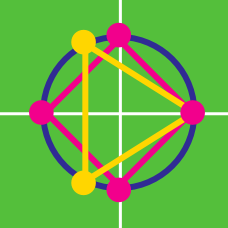Algebra

# Solving for Roots of Unity

What is the complex solution set of the equation $z^4-1=0$?

Which of these is a solution to the equation $z^6=12$?

Let $U_n$ denote the set of all $n^\text{th}$ roots of unity.

If $U_a=\{yz\mid y\in U_3,\ z\in U_5\}$, then $a=\ ?$

Which of these is a solution to the following equation?

$x^5+x^4+x^3+x^2+x+1=0$

If $a+bi$ is an $n^\text{th}$ root of unity, then which of these is not necessarily an $n^\text{th}$ root of unity?

×